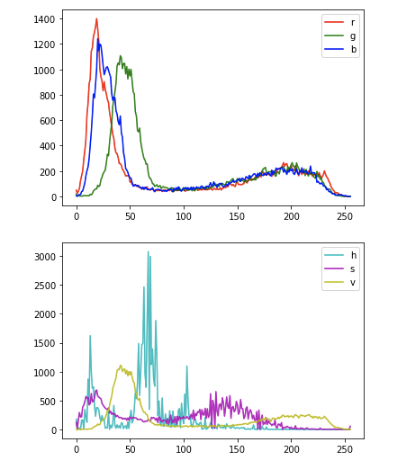# Introduction

I am dealing with images and wanted to use the RGB and HSV histogram information as features. So I tried to extract the histogram using ** OpenCV **. I will leave it as a memo.

# histogram

A `histogram can be thought of as a graph or plot to know the overall distribution of pixel values in an image. Generally, the horizontal axis is the pixel value, and the vertical axis is the frequency of appearance of the pixel value. Histogram visualization is one way to understand an image. `

# What is the difference between RGB and HSV?

RGB

RGB is an acronym for red, green, and blue, respectively. These three colors are called the "three primary colors of light," and can be used on cathode ray tubes, liquid crystal monitors, etc. It is a method for expressing. Since 256 levels of darkness can be set for each primary color, 16,777,216 colors of 256 cubes can be expressed.

HSV

HSV is a method of expressing color with three elements: hue (Hue), saturation (Saturation), and brightness (Value / Brightness). ** Hue **: Hue ring (hue ring) The shape of the ring or displayed in the range of 0 to 360 degrees ** Saturation **: Degree of vividness of color: The lower the value, the lower the saturation, and the higher the value, the higher the saturation. ** Brightness **: Degree of brightness of color: When the value is low, it becomes dark, and when it is high, it becomes bright.

Here are some points to note when loading images.

``````import cv2

img = cv2.imread("sample.jpg ")
``````

In this case, img is `BGR`. If you want to convert to `RGB`, you can convert it by the following method.

``````img_rgb = cv2.cvtColor(img, cv2.COLOR_BGR2RGB)
``````

``````from PIL import Image

img = Image.open("sample.jpg ")
``````

When reading with pillow, you can read with `RGB`.

# Function that returns RGB and HSV by specifying an image

I implemented a function that returns a histogram of R, G, B, H, S, V when an image is passed in Python. I also posted the result of executing with jupyter.

``````
def show_img(path):

b, g, r = img[:,:,0], img[:,:,1], img[:,:,2]
hist_b = cv2.calcHist([b],,None,,[0,256])
hist_g = cv2.calcHist([g],,None,,[0,256])
hist_r = cv2.calcHist([r],,None,,[0,256])
plt.plot(hist_r, color='r', label="r")
plt.plot(hist_g, color='g', label="g")
plt.plot(hist_b, color='b', label="b")
plt.legend()
plt.show()
img2 = cv2.cvtColor(img, cv2.COLOR_BGR2HSV)
h, s, v = img2[:,:,0], img2[:,:,1], img2[:,:,2]
hist_h = cv2.calcHist([h],,None,,[0,256])
hist_s = cv2.calcHist([s],,None,,[0,256])
hist_v = cv2.calcHist([v],,None,,[0,256])
plt.plot(hist_h, color='r', label="h")
plt.plot(hist_s, color='g', label="s")
plt.plot(hist_v, color='b', label="v")
plt.legend()
plt.show()

return hist_r,hist_g, hist_b, hist_h, hist_s, hist_v
``````

This time I will try with the following dog image.``````r,g,b,h,s,v = show_img("dog.jpeg ")
``````#### About histogram calculation

OpenCV uses the following functions to calculate the histogram.

`cv2.calcHist(images, channels, mask, histSize, ranges[, hist[, accumulate]])`

** images **: Input images. The pixel value data type is either uint8 or float32. Use the symbol [] to specify something like “[img]”. ** channels **: Index of the channel of the image for which the histogram is calculated. If the input image is a graceful image, specify . For color images, specify one of the values , , and  that corresponds to the hues of B, G, and R for which the histogram is calculated. ** mask **: This is a mask image. Specify “None” to calculate the histogram of all pixels in the image. To calculate the histogram of a specific area in the image, specify a mask image that represents the specific area (an example is shown below). ** histSize **: The number of bins. This argument is also specified using the symbol []. Specify  if you want to target all pixel values. ** ranges **: RANGE that represents the range of pixel values for which you want to measure the histogram. Normally, specify [0,256].

# References

-[Histogram # 1: Calculate, plot, and analyze !!!](http://labs.eecs.tottori-u.ac.jp/sd/Member/oyamada/OpenCV/html/py_tutorials/py_imgproc /py_histograms/py_histogram_begins/py_histogram_begins.html)